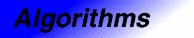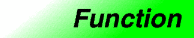set_unionCategory: algorithms Component type: function

Prototype

Set_union is an overloaded name; there are actually two set_union functions.

template <class InputIterator1, class InputIterator2, class OutputIterator>
OutputIterator set_union(InputIterator1 first1, InputIterator1 last1,
InputIterator2 first2, InputIterator2 last2,
OutputIterator result);

template <class InputIterator1, class InputIterator2, class OutputIterator,
class StrictWeakOrdering>
OutputIterator set_union(InputIterator1 first1, InputIterator1 last1,
InputIterator2 first2, InputIterator2 last2,
OutputIterator result,
StrictWeakOrdering comp);

Description

Set_union constructs a sorted range that is the union of the sorted ranges [first1, last1) and [first2, last2). The return value is the end of the output range.

In the simplest case, set_union performs the "union" operation from set theory: the output range contains a copy of every element that is contained in [first1, last1), [first2, last2), or both. The general case is more complicated, because the input ranges may contain duplicate elements. The generalization is that if a value appears m times in [first1, last1) and n times in [first2, last2) (where m or n may be zero), then it appears max(m,n) times in the output range.  Set_union is stable, meaning both that the relative order of elements within each input range is preserved, and that if an element is present in both input ranges it is copied from the first range rather than the second.

The two versions of set_union differ in how they define whether one element is less than another. The first version compares objects using operator<, and the second compares objects using a functors comp.

Definition

Defined in the standard header algorithm, and in the nonstandard backward-compatibility header algo.h.

Requirements on types

For the first version:

• InputIterator1 is a model of InputIterator.
• InputIterator2 is a model of InputIterator.
• OutputIterator is a model of OutputIterator.
• InputIterator1 and InputIterator2 have the same value type.
• InputIterator's value type is a model of LessThanComparable.
• The ordering on objects of InputIterator1's value type is a strict weak ordering, as defined in the LessThanComparable requirements.
• InputIterator's value type is convertible to a type in OutputIterator's set of value types.

For the second version:

• InputIterator1 is a model of InputIterator.
• InputIterator2 is a model of InputIterator.
• OutputIterator is a model of OutputIterator.
• StrictWeakOrdering is a model of StrictWeakOrdering.
• InputIterator1 and InputIterator2 have the same value type.
• InputIterator1's value type is convertible to StrictWeakOrdering's argument type.
• InputIterator's value type is convertible to a type in OutputIterator's set of value types.

Preconditions

For the first version:

• [first1, last1) is a valid range.
• [first2, last2) is a valid range.
• [first1, last1) is ordered in ascending order according to operator<. That is, for every pair of iterators i and j in [first1, last1) such that i precedes j, *j < *i is false.
• [first2, last2) is ordered in ascending order according to operator<. That is, for every pair of iterators i and j in [first2, last2) such that i precedes j, *j < *i is false.
• There is enough space to hold all of the elements being copied. More formally, the requirement is that [result, result + n) is a valid range, where n is the number of elements in the union of the two input ranges.
• [first1, last1) and [result, result + n) do not overlap.
• [first2, last2) and [result, result + n) do not overlap.

For the second version:

• [first1, last1) is a valid range.
• [first2, last2) is a valid range.
• [first1, last1) is ordered in ascending order according to comp. That is, for every pair of iterators i and j in [first1, last1) such that i precedes j, comp(*j, *i) is false.
• [first2, last2) is ordered in ascending order according to comp. That is, for every pair of iterators i and j in [first2, last2) such that i precedes j, comp(*j, *i) is false.
• There is enough space to hold all of the elements being copied. More formally, the requirement is that [result, result + n) is a valid range, where n is the number of elements in the union of the two input ranges.
• [first1, last1) and [result, result + n) do not overlap.
• [first2, last2) and [result, result + n) do not overlap.

Complexity

Linear. Zero comparisons if either [first1, last1) or [first2, last2) is empty, otherwise at most 2 * ((last1 - first1) + (last2 - first2)) - 1 comparisons.

Example

inline bool lt_nocase(char c1, char c2) { return tolower(c1) < tolower(c2); }

int main()
{
int A1[] = {1, 3, 5, 7, 9, 11};
int A2[] = {1, 1, 2, 3, 5, 8, 13};
char A3[] = {'a', 'b', 'B', 'B', 'f', 'H'};
char A4[] = {'A', 'B', 'b', 'C', 'D', 'F', 'F', 'h', 'h'};

const int N1 = sizeof(A1) / sizeof(int);
const int N2 = sizeof(A2) / sizeof(int);
const int N3 = sizeof(A3);
const int N4 = sizeof(A4);

cout << "Union of A1 and A2: ";
set_union(A1, A1 + N1, A2, A2 + N2,
ostream_iterator<int>(cout, " "));
cout << endl
<< "Union of A3 and A4: ";
set_union(A3, A3 + N3, A4, A4 + N4,
ostream_iterator<char>(cout, " "),
lt_nocase);
cout << endl;
}

The output is

Union of A1 and A2: 1 1 2 3 5 7 8 9 11 13
Union of A3 and A4: a b B B C D f F H h